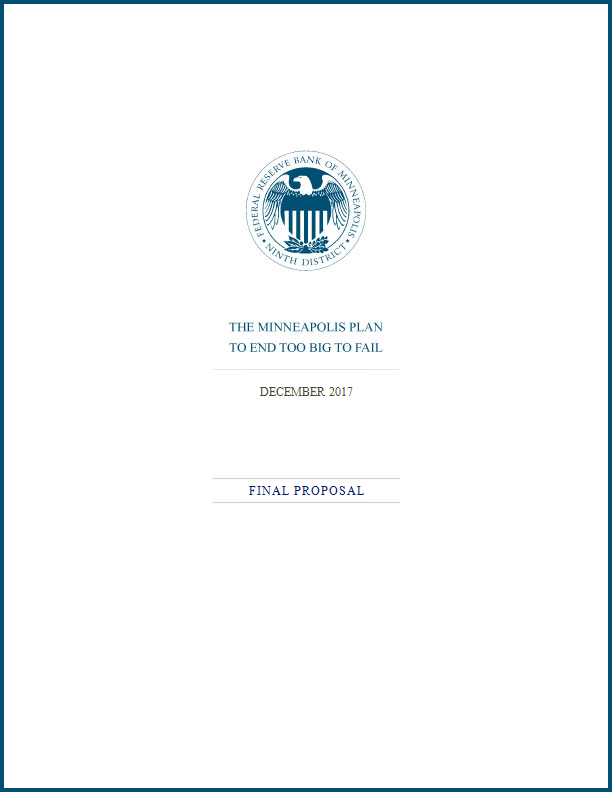We serve the public by pursuing a growing economy and stable financial system that work for all of us.

### Region & Community

We examine economic issues that deeply affect our communities.

### Research

We conduct world-class research to inform and inspire policymakers and the public.

### Banking

We provide the banking community with timely information and useful guidance.

### Policy

We explore policy topics that are important for advancing prosperity across our region.

# Appendix A: The Leverage Ratio in the Minneapolis Plan### Read the full plan [PDF]

A primary recommendation of the Minneapolis Plan is setting a minimum equity capital requirement for covered banks at 23.5 percent. This figure measures equity capital as a share of risk-weighted assets. We note that the equivalent leverage ratio is 15 percent of total assets.

In this appendix, we explain why we recommend a leverage ratio target and how we calculate it.

We recommend the use of a corresponding leverage ratio target to avoid cases where the risk weights assigned to a given asset type are too low. In those cases, the amount of equity a bank funds itself with relative to its underlying risk would also be too low, and the bank would pose a higher chance of failure, all else equal. We do not rely exclusively on a leverage ratio because that approach treats all assets as equally risky and thus potentially does not align capital with bank risk-taking.

We derived the 15 percent proposed leverage ratio that corresponds to our proposed risk-weighted asset capital ratio recommendation with a simple transformation. First, we calculated the average relationship between the total assets that covered banks report in their leverage ratio and the risk-weighted assets they report. Second, we used that estimate to convert our 23.5 percent risk-weighted asset capital ratio to the simple leverage ratio. We used measures of on-balance-sheet assets in this calculation.

Specifically, we calculated that total assets for covered banks were 1.6 times risk-weighted assets using year-end 2015 data. We divided 23.5 by 1.6 to come to the corresponding leverage ratio of 14.6, which we rounded to 15.

Banks also have what are called “off-balance-sheet” assets. For example, a bank may enter into a financial contract that could expose the bank to future loss just as traditional loans do. Under certain conditions, the bank may not have to account for this contract as an on-balance-sheet asset in the current period. There are leverage ratio measures that include these off-balance-sheet exposures as a part of total assets in the calculation.

If we had used measures of total assets that accounted for off-balance-sheet assets in our calculation, the leverage ratio equivalent to 23.5 percent would have been 13 percent, lower than 15 percent. Why? Because total asset measures that account for off-balance-sheet assets, usually referred to as “total exposures,” are larger than the total asset measures that do not.

We calculate a simple leverage ratio that does not account for off-balance-sheet exposures because:

• There is no one single “correct” leverage ratio. There are multiple legitimate measures. Throughout our analysis, we have used the simplest approach when we have multiple, reasonable options. We use the basic approach in this case as well.
• Many important uses of capital measures continue to use a simple leverage ratio, which is based on the on-balance-sheet asset approach. For example, we believe the “stress test” run by the Federal Reserve System is a particularly important supervisory exercise for the most systemically important banks. The stress test continues to use a simple leverage ratio measure, among others, to assess the post-stress capital position of banks.
• The broader measures of leverage based on total exposures also typically allow for certain non-equity forms of capital to count in the numerator of the ratio. We oppose such inclusion.
• The data to convert our risk-weighted target to a leverage target using the broad measure of assets were not available for inclusion in the November 2016 draft Plan.